## Problem Set: The Fundamental Theorem of Calculus

1. Consider two athletes running at variable speeds ${v}_{1}(t)$ and ${v}_{2}(t).$ The runners start and finish a race at exactly the same time. Explain why the two runners must be going the same speed at some point.

2. Two mountain climbers start their climb at base camp, taking two different routes, one steeper than the other, and arrive at the peak at exactly the same time. Is it necessarily true that, at some point, both climbers increased in altitude at the same rate?

3. To get on a certain toll road a driver has to take a card that lists the mile entrance point. The card also has a timestamp. When going to pay the toll at the exit, the driver is surprised to receive a speeding ticket along with the toll. Explain how this can happen.

4. Set $F(x)={\displaystyle\int }_{1}^{x}(1-t)dt.$ Find ${F}^{\prime }(2)$ and the average value of ${F}^{\text{′}}$ over $\left[1,2\right].$

In the following exercises, use the Fundamental Theorem of Calculus, Part 1, to find each derivative.

5. $\frac{d}{dx}{\displaystyle\int }_{1}^{x}{e}^{\text{−}{t}^{2}}dt$

6. $\frac{d}{dx}{\displaystyle\int }_{1}^{x}{e}^{ \cos t}dt$

7. $\frac{d}{dx}{\displaystyle\int }_{3}^{x}\sqrt{9-{y}^{2}}dy$

8. $\frac{d}{dx}{\displaystyle\int }_{4}^{x}\frac{ds}{\sqrt{16-{s}^{2}}}$

9. $\frac{d}{dx}{\displaystyle\int }_{x}^{2x}tdt$

10. $\frac{d}{dx}{\displaystyle\int }_{0}^{\sqrt{x}}tdt$

11. $\frac{d}{dx}{\displaystyle\int }_{0}^{ \sin x}\sqrt{1-{t}^{2}}dt$

12. $\frac{d}{dx}{\displaystyle\int }_{ \cos x}^{1}\sqrt{1-{t}^{2}}dt$

13. $\frac{d}{dx}{\displaystyle\int }_{1}^{\sqrt{x}}\frac{{t}^{2}}{1+{t}^{4}}dt$

14. $\frac{d}{dx}{\displaystyle\int }_{1}^{{x}^{2}}\frac{\sqrt{t}}{1+t}dt$

15. $\frac{d}{dx}{\displaystyle\int }_{0}^{\text{ln}x}{e}^{t}dt$

16. $\frac{d}{dx}{\displaystyle\int }_{1}^{{e}^{2}}\text{ln}{u}^{2}du$

17. The graph of $y={\displaystyle\int }_{0}^{x}f(t)dt,$ where $f$ is a piecewise constant function, is shown here.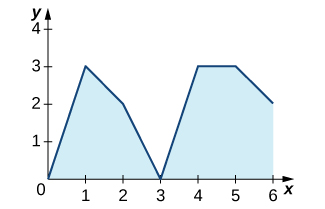1. Over which intervals is $f$ positive? Over which intervals is it negative? Over which intervals, if any, is it equal to zero?
2. What are the maximum and minimum values of $f$?
3. What is the average value of $f$?

18. The graph of $y={\displaystyle\int }_{0}^{x}f(t)dt,$ where $f$ is a piecewise constant function, is shown here.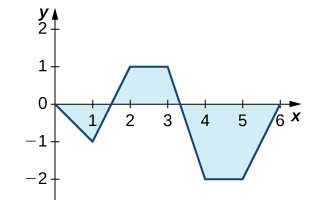1. Over which intervals is $f$ positive? Over which intervals is it negative? Over which intervals, if any, is it equal to zero?
2. What are the maximum and minimum values of $f$?
3. What is the average value of $f$?

19. The graph of $y={\displaystyle\int }_{0}^{x}\ell (t)dt,$ where is a piecewise linear function, is shown here.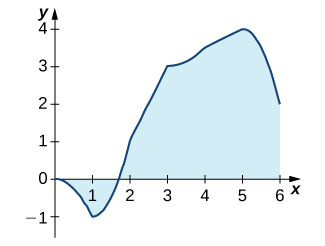1. Over which intervals is positive? Over which intervals is it negative? Over which, if any, is it zero?
2. Over which intervals is increasing? Over which is it decreasing? Over which, if any, is it constant?
3. What is the average value of ?

20. The graph of $y={\displaystyle\int }_{0}^{x}\ell (t)dt,$ where is a piecewise linear function, is shown here.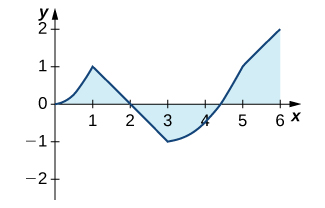1. Over which intervals is positive? Over which intervals is it negative? Over which, if any, is it zero?
2. Over which intervals is increasing? Over which is it decreasing? Over which intervals, if any, is it constant?
3. What is the average value of ?

In the following exercises, use a calculator to estimate the area under the curve by computing T10, the average of the left- and right-endpoint Riemann sums using $N=10$ rectangles. Then, using the Fundamental Theorem of Calculus, Part 2, determine the exact area.

21. [T] $y={x}^{2}$ over $\left[0,4\right]$

22. [T] $y={x}^{3}+6{x}^{2}+x-5$ over $\left[-4,2\right]$

23. [T] $y=\sqrt{{x}^{3}}$ over $\left[0,6\right]$

24. [T] $y=\sqrt{x}+{x}^{2}$ over $\left[1,9\right]$

25. [T] $\displaystyle\int ( \cos x- \sin x)dx$ over $\left[0,\pi \right]$

26. [T] $\displaystyle\int \frac{4}{{x}^{2}}dx$ over $\left[1,4\right]$

In the following exercises, evaluate each definite integral using the Fundamental Theorem of Calculus, Part 2.

27. ${\displaystyle\int }_{-1}^{2}({x}^{2}-3x)dx$

28. ${\displaystyle\int }_{-2}^{3}({x}^{2}+3x-5)dx$

29. ${\displaystyle\int }_{-2}^{3}(t+2)(t-3)dt$

30. ${\displaystyle\int }_{2}^{3}({t}^{2}-9)(4-{t}^{2})dt$

31. ${\displaystyle\int }_{1}^{2}{x}^{9}dx$

32. ${\displaystyle\int }_{0}^{1}{x}^{99}dx$

33. ${\displaystyle\int }_{4}^{8}(4{t}^{5\text{/}2}-3{t}^{3\text{/}2})dt$

34. ${\displaystyle\int }_{1\text{/}4}^{4}({x}^{2}-\frac{1}{{x}^{2}})dx$

35. ${\displaystyle\int }_{1}^{2}\frac{2}{{x}^{3}}dx$

36. ${\displaystyle\int }_{1}^{4}\frac{1}{2\sqrt{x}}dx$

37. ${\displaystyle\int }_{1}^{4}\frac{2-\sqrt{t}}{{t}^{2}}dt$

38. ${\displaystyle\int }_{1}^{16}\frac{dt}{{t}^{1\text{/}4}}$

39. ${\displaystyle\int }_{0}^{2\pi } \cos \theta d\theta$

40. ${\displaystyle\int }_{0}^{\pi \text{/}2} \sin \theta d\theta$

41. ${\displaystyle\int }_{0}^{\pi \text{/}4}{ \sec }^{2}\theta d\theta$

42. ${\displaystyle\int }_{0}^{\pi \text{/}4} \sec \theta \tan {\theta}d\theta$

43. ${\displaystyle\int }_{\pi \text{/}3}^{\pi \text{/}4} \csc \theta \cot \theta d\theta$

44. ${\displaystyle\int }_{\pi \text{/}4}^{\pi \text{/}2}{ \csc }^{2}\theta d\theta$

45. ${\displaystyle\int }_{1}^{2}(\frac{1}{{t}^{2}}-\frac{1}{{t}^{3}})dt$

46. ${\displaystyle\int }_{-2}^{-1}(\frac{1}{{t}^{2}}-\frac{1}{{t}^{3}})dt$

In the following exercises, use the evaluation theorem to express the integral as a function $F(x).$

47. ${\displaystyle\int }_{a}^{x}{t}^{2}dt$

48. ${\displaystyle\int }_{1}^{x}{e}^{t}dt$

49. ${\displaystyle\int }_{0}^{x} \cos tdt$

50. ${\displaystyle\int }_{\text{−}x}^{x} \sin tdt$

In the following exercises, identify the roots of the integrand to remove absolute values, then evaluate using the Fundamental Theorem of Calculus, Part 2.

51. ${\displaystyle\int }_{-2}^{3}|x|dx$

52. ${\displaystyle\int }_{-2}^{4}|{t}^{2}-2t-3|dt$

53. ${\displaystyle\int }_{0}^{\pi }| \cos t|dt$

54. ${\displaystyle\int }_{\text{−}\pi \text{/}2}^{\pi \text{/}2}| \sin t|dt$

55. Suppose that the number of hours of daylight on a given day in Seattle is modeled by the function $-3.75 \cos (\frac{\pi t}{6})+12.25,$ with $t$ given in months and $t=0$ corresponding to the winter solstice.

1. What is the average number of daylight hours in a year?
2. At which times $t$1 and $t$2, where $0\le {t}_{1}<{t}_{2}<12,$ do the number of daylight hours equal the average number?
3. Write an integral that expresses the total number of daylight hours in Seattle between ${t}_{1}$ and ${t}_{2}.$
4. Compute the mean hours of daylight in Seattle between ${t}_{1}$ and ${t}_{2},$ where $0\le {t}_{1}<{t}_{2}<12,$ and then between ${t}_{2}$ and ${t}_{1},$ and show that the average of the two is equal to the average day length.

56. Suppose the rate of gasoline consumption in the United States can be modeled by a sinusoidal function of the form $(11.21- \cos (\frac{\pi t}{6}))×{10}^{9}$ gal/mo.

1. What is the average monthly consumption, and for which values of $t$ is the rate at time $t$ equal to the average rate?
2. What is the number of gallons of gasoline consumed in the United States in a year?
3. Write an integral that expresses the average monthly U.S. gas consumption during the part of the year between the beginning of April $(t=3)$ and the end of September $(t=9\text{).}$

57. Explain why, if $f$ is continuous over $\left[a,b\right],$ there is at least one point $c\in \left[a,b\right]$ such that $f(c)=\frac{1}{b-a}{\displaystyle\int }_{a}^{b}f(t)dt.$

58. Explain why, if $f$ is continuous over $\left[a,b\right]$ and is not equal to a constant, there is at least one point $M\in \left[a,b\right]$ such that $f(M)=\frac{1}{b-a}{\displaystyle\int }_{a}^{b}f(t)dt$ and at least one point $m\in \left[a,b\right]$ such that $f(m)<\frac{1}{b-a}{\displaystyle\int }_{a}^{b}f(t)dt.$

59. Kepler’s first law states that the planets move in elliptical orbits with the Sun at one focus. The closest point of a planetary orbit to the Sun is called the perihelion (for Earth, it currently occurs around January 3) and the farthest point is called the aphelion (for Earth, it currently occurs around July 4). Kepler’s second law states that planets sweep out equal areas of their elliptical orbits in equal times. Thus, the two arcs indicated in the following figure are swept out in equal times. At what time of year is Earth moving fastest in its orbit? When is it moving slowest?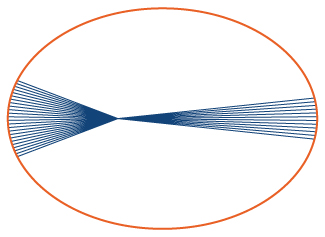60. A point on an ellipse with major axis length 2$a$ and minor axis length 2$b$ has the coordinates $(a \cos \theta ,b \sin \theta ),0\le \theta \le 2\pi .$

1. Show that the distance from this point to the focus at $(\text{−}c,0)$ is $d(\theta )=a+c \cos \theta ,$ where $c=\sqrt{{a}^{2}-{b}^{2}}.$
2. Use these coordinates to show that the average distance $\overline{d}$ from a point on the ellipse to the focus at $(\text{−}c,0),$ with respect to angle θ, is $a$.

61. As implied earlier, according to Kepler’s laws, Earth’s orbit is an ellipse with the Sun at one focus. The perihelion for Earth’s orbit around the Sun is 147,098,290 km and the aphelion is 152,098,232 km.

1. By placing the major axis along the $x$-axis, find the average distance from Earth to the Sun.
2. The classic definition of an astronomical unit (AU) is the distance from Earth to the Sun, and its value was computed as the average of the perihelion and aphelion distances. Is this definition justified?

62. The force of gravitational attraction between the Sun and a planet is $F(\theta )=\frac{GmM}{{r}^{2}(\theta )},$ where $m$ is the mass of the planet, M is the mass of the Sun, G is a universal constant, and $r(\theta )$ is the distance between the Sun and the planet when the planet is at an angle θ with the major axis of its orbit. Assuming that M, $m$, and the ellipse parameters $a$ and $b$ (half-lengths of the major and minor axes) are given, set up—but do not evaluate—an integral that expresses in terms of $G,m,M,a,b$ the average gravitational force between the Sun and the planet.

63. The displacement from rest of a mass attached to a spring satisfies the simple harmonic motion equation $x(t)=A \cos (\omega t-\varphi ),$ where $\varphi$ is a phase constant, ω is the angular frequency, and A is the amplitude. Find the average velocity, the average speed (magnitude of velocity), the average displacement, and the average distance from rest (magnitude of displacement) of the mass.